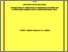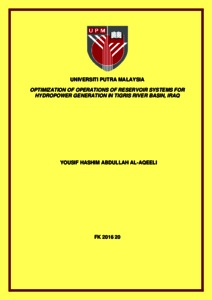# Optimization of operations of reservoir systems for hydropower generation in Tigris River Basin, Iraq

## Citation

Al-Aqeel, Yousif Hashim Abdullah (2016) Optimization of operations of reservoir systems for hydropower generation in Tigris River Basin, Iraq. Doctoral thesis, Universiti Putra Malaysia.

## Abstract

The aim of the study was to determine the optimal operation policies for the various combinations of up to four reservoirs (Mosul and Dokan reservoirs are currently in operation, Bekhma reservoir is under construction while Makhoul reservoir is under planning phase) along the Tigris river basin in Iraq for the purpose of optimally generating hydroelectric power, given the constraints pertinent to the area. A genetic algorithm optimization model (GAOM) was coded in the Matlab work environment to determine the optimal operating policy for a single reservoir to maximize the annual hydropower generation. Two algorithms were next formulated and used independently in the GAOM to improve the performance of the Mosul reservoir in Iraq, during 20 years of operation. The performances of these two algorithms were evaluated through comparing their optimal values. The GAOM achieved an increase in hydropower generation in 17 years of the 20 years with the second algorithm being the better. To determine the effect of precipitation and evaporation, their volumes were taken into consideration in the continuity equation, using the second algorithm. In this case, the obtained optimal values were decreased in all of the scenarios compared with ignoring the volumes of precipitation and evaporation. A simulation model (SM) for the Mosul reservoir was formulated by using the SIMULINK technique in the MATLAB environment. The r2 and NSE are 0.92 and 0.87 respectively in comparison of elevations, and 0.81 and 0.77 respectively in comparisons of hydropower generated. Adopting the second algorithm and taking into consideration the evaporation and precipitation, the GAOM and SM of Mosul reservoir were combined. In this combination, the GAOM identified the optimal operation policy, while the SM adopted this optimal operation policy to evaluate the performance of GAOM calculations through comparing the three parameters; powers, elevations and storages in order to confirm the calculations of GAOM. In all of these comparisons, the coefficient of determination was 0.99.Two operational strategies in the combination of GAOMs and SMs were used to determine the optimal operation policies for the multi-reservoir system located on the Tigris river basin in Iraq. In the first strategy, the target storage at the end of final month is equal to, or above the minimum operational storage; and for the second strategy it is equal to, or above the initial storage. For each strategy, the performances of operation the reservoirs individually; as a dual-reservoir system; as a three-reservoir system and as a four-reservoir system were compared in terms of annual hydropower generation based on the three groups of annual inflows: minimum, average, and maximum during twenty years. Those operational situations where: firstly, running of Mosul and Dokan reservoirs, secondly, running of Mosul, Dokan and Bekhma reservoirs, and thirdly, running of Mosul, Dokan, Bekhma and Makhoul reservoirs. According to the values of T-test obtained, the results indicated that only using the first strategy and operating Mosul, Dokan, Bekhma and Makhoul reservoirs were significant in favor of running the reservoirs as one entity. The two strategies mentioned previously were also used in the combined GAOMs and SMs to determine the optimal operation policies for the multi-reservoir system in the case of using a new storage of Makhoul reservoir. For each strategy, the performance of the operation of the multi-reservoir system was assessed through comparing the annual hydropower production a prior to and after having used this new storage by relying on three modes of annual inflows, minimum, average, and maximum annual inflows. All of these comparisons were done in two cases: operating the reservoirs individually and as one system. Through using the first and second strategies, the hydropower production increased by good percentages through using those three modes of annual inflows. In addition, for each strategy, the performance of this multi-reservoir system was evaluated according to that new storage of Makhoul. In this evaluation, the annual hydropower production by operating the reservoirs individually and as one system were compared by using those three modes of inflows according to T-test. The results indicated that the differences between the hydropower generated were significant in favor of operating the reservoirs as one system through using the first strategy, while were non-significant by using the second strategy. The parameters of evaporation and precipitation must include in the continuity equation when using or creating a model simulates the dynamic processes of water storage system. Hybrid model, consists of GAOM and SM, was created. This model has high reliability and can be used in real applications with various storage systems.Keywords: reservoir systems, optimization, simulation, optimal operation policy, genetic algorithms, Matlab, Simulink, hydropower generation, Tigris river basin, Iraq.Preview
Text
FK 2016 20 IR.pdfView Item